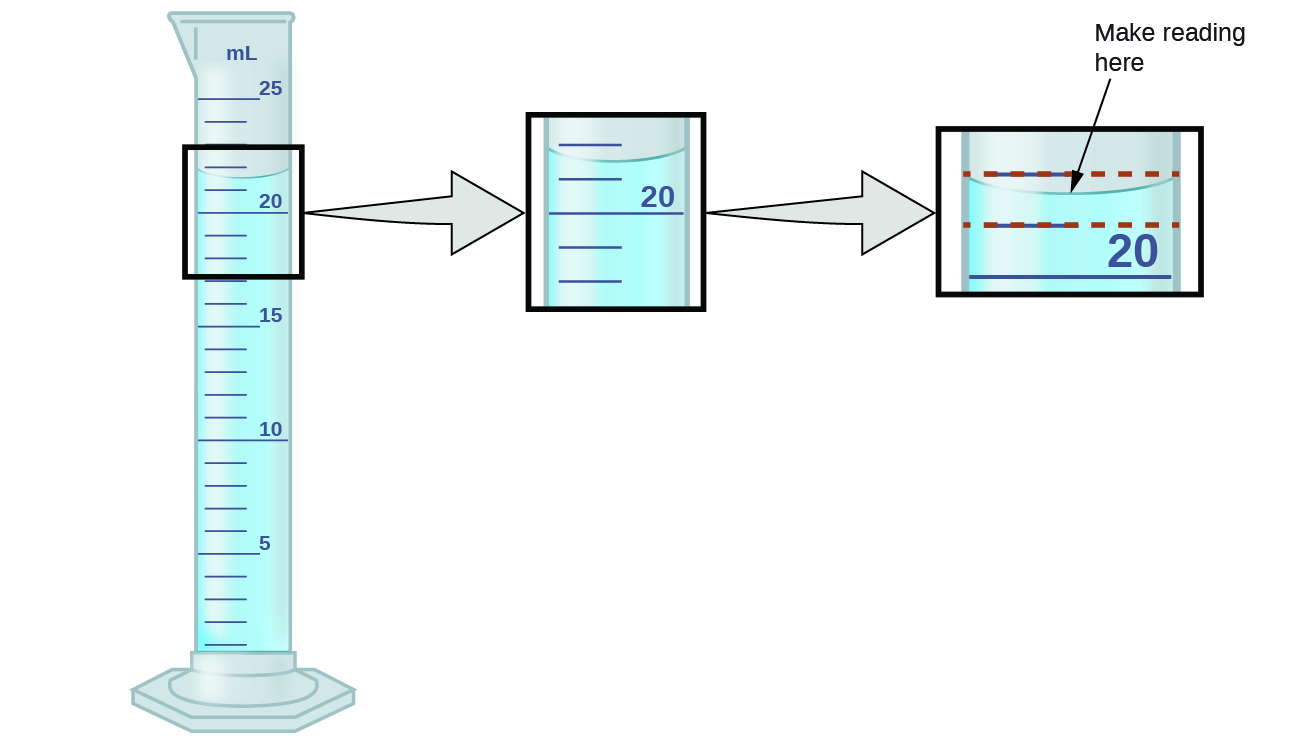1.5 Measurement uncertainty, accuracy, and precision

 Page 1 / 11
By the end of this section, you will be able to:
• Define accuracy and precision
• Distinguish exact and uncertain numbers
• Correctly represent uncertainty in quantities using significant figures
• Apply proper rounding rules to computed quantities

Counting is the only type of measurement that is free from uncertainty, provided the number of objects being counted does not change while the counting process is underway. The result of such a counting measurement is an example of an exact number    . If we count eggs in a carton, we know exactly how many eggs the carton contains. The numbers of defined quantities are also exact. By definition, 1 foot is exactly 12 inches, 1 inch is exactly 2.54 centimeters, and 1 gram is exactly 0.001 kilogram. Quantities derived from measurements other than counting, however, are uncertain to varying extents due to practical limitations of the measurement process used.

Significant figures in measurement

The numbers of measured quantities, unlike defined or directly counted quantities, are not exact. To measure the volume of liquid in a graduated cylinder, you should make a reading at the bottom of the meniscus, the lowest point on the curved surface of the liquid.To measure the volume of liquid in this graduated cylinder, you must mentally subdivide the distance between the 21 and 22 mL marks into tenths of a milliliter, and then make a reading (estimate) at the bottom of the meniscus.

Refer to the illustration in [link] . The bottom of the meniscus in this case clearly lies between the 21 and 22 markings, meaning the liquid volume is certainly greater than 21 mL but less than 22 mL. The meniscus appears to be a bit closer to the 22-mL mark than to the 21-mL mark, and so a reasonable estimate of the liquid’s volume would be 21.6 mL. In the number 21.6, then, the digits 2 and 1 are certain, but the 6 is an estimate. Some people might estimate the meniscus position to be equally distant from each of the markings and estimate the tenth-place digit as 5, while others may think it to be even closer to the 22-mL mark and estimate this digit to be 7. Note that it would be pointless to attempt to estimate a digit for the hundredths place, given that the tenths-place digit is uncertain. In general, numerical scales such as the one on this graduated cylinder will permit measurements to one-tenth of the smallest scale division. The scale in this case has 1-mL divisions, and so volumes may be measured to the nearest 0.1 mL.

This concept holds true for all measurements, even if you do not actively make an estimate. If you place a quarter on a standard electronic balance, you may obtain a reading of 6.72 g. The digits 6 and 7 are certain, and the 2 indicates that the mass of the quarter is likely between 6.71 and 6.73 grams. The quarter weighs about 6.72 grams, with a nominal uncertainty in the measurement of ± 0.01 gram. If we weigh the quarter on a more sensitive balance, we may find that its mass is 6.723 g. This means its mass lies between 6.722 and 6.724 grams, an uncertainty of 0.001 gram. Every measurement has some uncertainty    , which depends on the device used (and the user’s ability). All of the digits in a measurement, including the uncertain last digit, are called significant figures    or significant digits . Note that zero may be a measured value; for example, if you stand on a scale that shows weight to the nearest pound and it shows “120,” then the 1 (hundreds), 2 (tens) and 0 (ones) are all significant (measured) values.

What is stoichometry
what is atom
An indivisible part of an element
ngwuebo
the smallest particle of an element which is indivisible is called an atom
Aloaye
An atom is the smallest indivisible particle of an element that can take part in chemical reaction
Alieu
is carbonates soluble
what is the difference between light and electricity
What is atom? atom can be defined as the smallest particles
what is the difference between Anode and nodes?
What's the net equations for the three steps of dissociation of phosphoric acid?
what is chemistry
the study of matter
Reginald
what did the first law of thermodynamics say
energy can neither be created or distroyed it can only be transferred or converted from one form to another
Graham's law of Diffusion
what is melting vaporization
melting and boiling point explain in term of molecular motion and Brownian movement
Anieke
Scientific notation for 150.9433962
what is aromaticity
aromaticity is a conjugated pi system specific to organic rings like benzene, which have an odd number of electron pairs within the system that allows for exceptional molecular stability
Pookieman
what is caustic soda
sodium hydroxide (NaOH)
Kamaluddeen
what is distilled water
Rihanat
is simply means a condensed water vapour
Kamaluddeen
a hydrocarbon contains 7.7 percent by mass of hydrogen and 92.3 percent by mass of carbon
how many types of covalent r there
how many covalent bond r there
JArim
they are three 3
TYPES OF COVALENT BOND-POLAR BOND-NON POLAR BOND-DOUBLE BOND-TRIPPLE BOND. There are three types of covalent bond depending upon the number of shared electron pairs. A covalent bond formed by the mutual sharing of one electron pair between two atoms is called a "Single Covalent bond.
UsmanByByBy Mistry Bhavesh